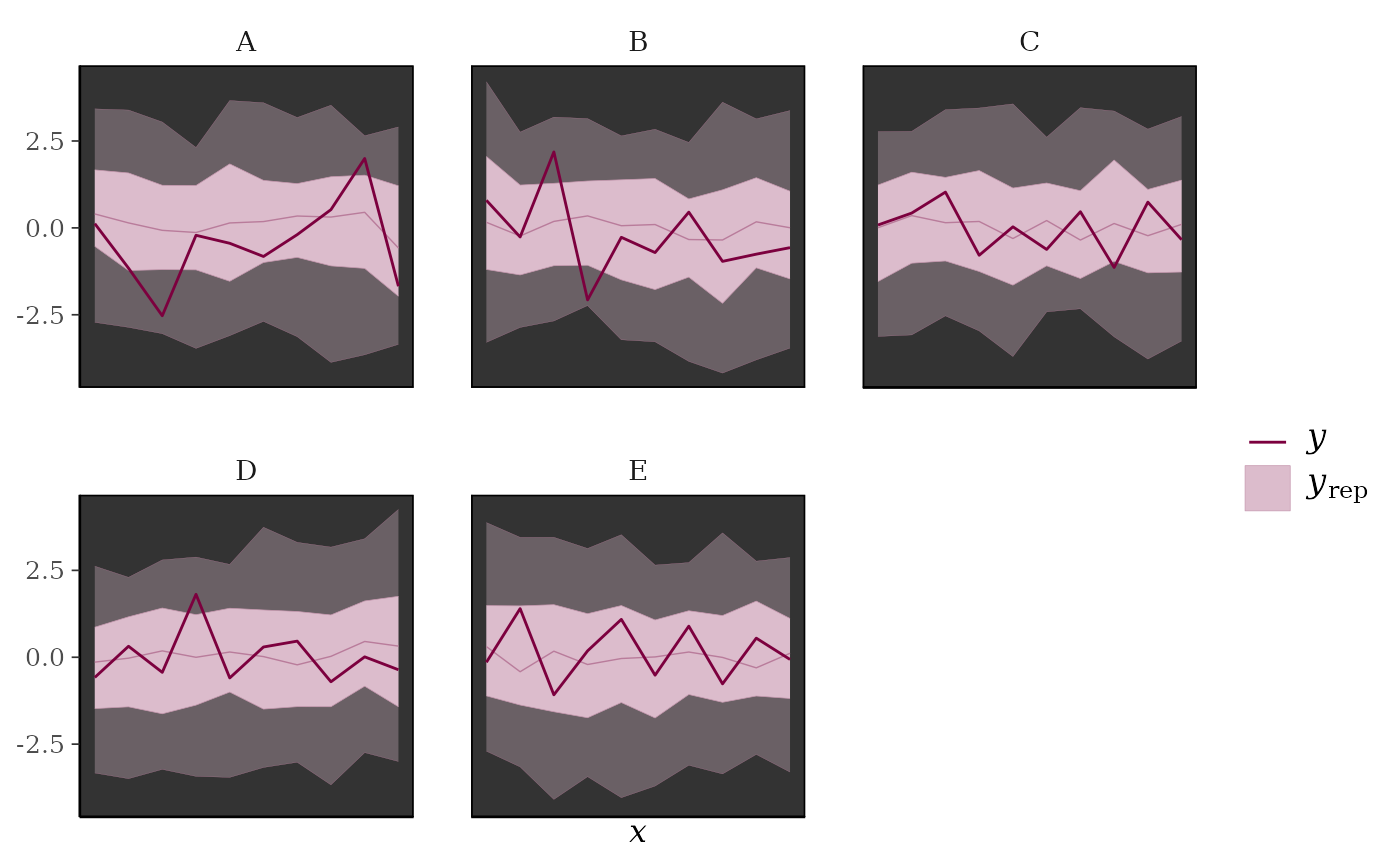Medians and central interval estimates of yrep with y overlaid. See the Plot Descriptions section, below.

ppc_intervals(y, yrep, x = NULL, ..., prob = 0.5, prob_outer = 0.9,
size = 1, fatten = 3)

ppc_intervals_grouped(y, yrep, x = NULL, group, ...,
facet_args = list(), prob = 0.5, prob_outer = 0.9, size = 1,
fatten = 3)

ppc_ribbon(y, yrep, x = NULL, ..., prob = 0.5, prob_outer = 0.9,
alpha = 0.33, size = 0.25)

ppc_ribbon_grouped(y, yrep, x = NULL, group, ..., facet_args = list(),
prob = 0.5, prob_outer = 0.9, alpha = 0.33, size = 0.25)

ppc_intervals_data(y, yrep, x = NULL, group = NULL, ..., prob = 0.5,
prob_outer = 0.9)

ppc_ribbon_data(y, yrep, x = NULL, group = NULL, ..., prob = 0.5,
prob_outer = 0.9)

## Arguments

y A vector of observations. See Details. An $$S$$ by $$N$$ matrix of draws from the posterior predictive distribution, where $$S$$ is the size of the posterior sample (or subset of the posterior sample used to generate yrep) and $$N$$ is the number of observations (the length of y). The columns of yrep should be in the same order as the data points in y for the plots to make sense. See Details for additional instructions. A numeric vector the same length as y to use as the x-axis variable. For example, x could be a predictor variable from a regression model, a time variable for time-series models, etc. If x is missing or NULL, then 1:length(y) is used for the x-axis. Currently unused. Values between 0 and 1 indicating the desired probability mass to include in the inner and outer intervals. The defaults are prob=0.5 and prob_outer=0.9. A grouping variable (a vector or factor) the same length as y. Each value in group is interpreted as the group level pertaining to the corresponding value of y. An optional list of arguments (other than facets) passed to ggplot2::facet_wrap() to control faceting. Arguments passed to geoms. For ribbon plots alpha and size are passed to ggplot2::geom_ribbon(). For interval plots size and fatten are passed to ggplot2::geom_pointrange().

## Value

The plotting functions return a ggplot object that can be further customized using the ggplot2 package. The functions with suffix _data() return the data that would have been drawn by the plotting function.

## Plot Descriptions

ppc_intervals(), ppc_ribbon()

100*prob% central intervals for yrep at each x value. ppc_intervals() plots intervals as vertical bars with points indicating yrep medians and darker points indicating observed y values. ppc_ribbon() plots a ribbon of connected intervals with a line through the median of yrep and a darker line connecting observed y values. In both cases an optional x variable can also be specified for the x-axis variable. Depending on the number of observations and the variability in the predictions at different values of x, one or the other of these plots may be easier to read than the other.

ppc_intervals_grouped(), ppc_ribbon_grouped()

Same as ppc_intervals() and ppc_ribbon(), respectively, but a separate plot (facet) is generated for each level of a grouping variable.

Gelman, A., Carlin, J. B., Stern, H. S., Dunson, D. B., Vehtari, A., and Rubin, D. B. (2013). Bayesian Data Analysis. Chapman & Hall/CRC Press, London, third edition. (Ch. 6)

## See also

Other PPCs: PPC-discrete, PPC-distributions, PPC-errors, PPC-loo, PPC-overview, PPC-scatterplots, PPC-test-statistics

## Examples

y <- rnorm(50)
yrep <- matrix(rnorm(5000, 0, 2), ncol = 50)

color_scheme_set("brightblue")
ppc_ribbon(y, yrep)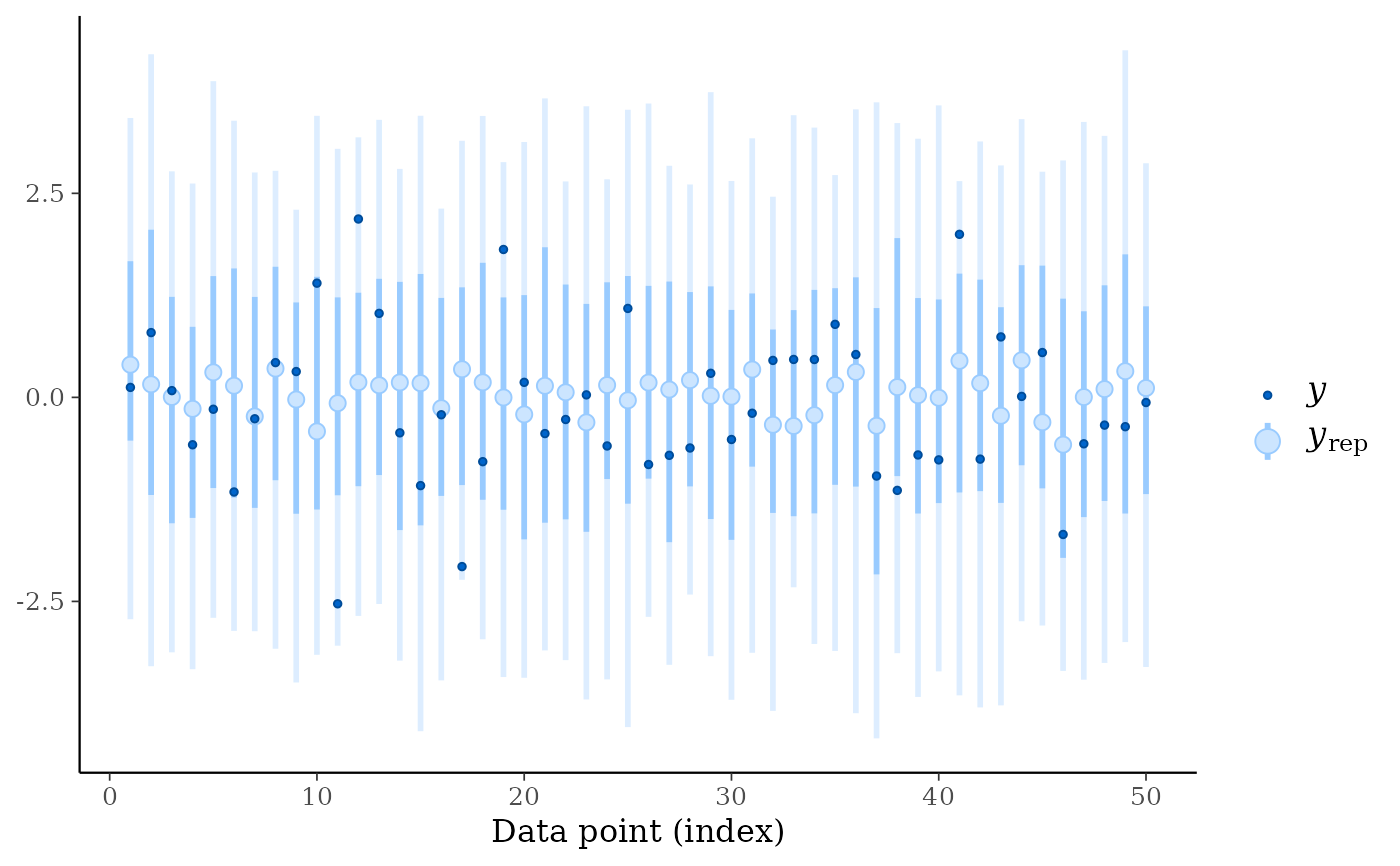ppc_intervals(y, yrep)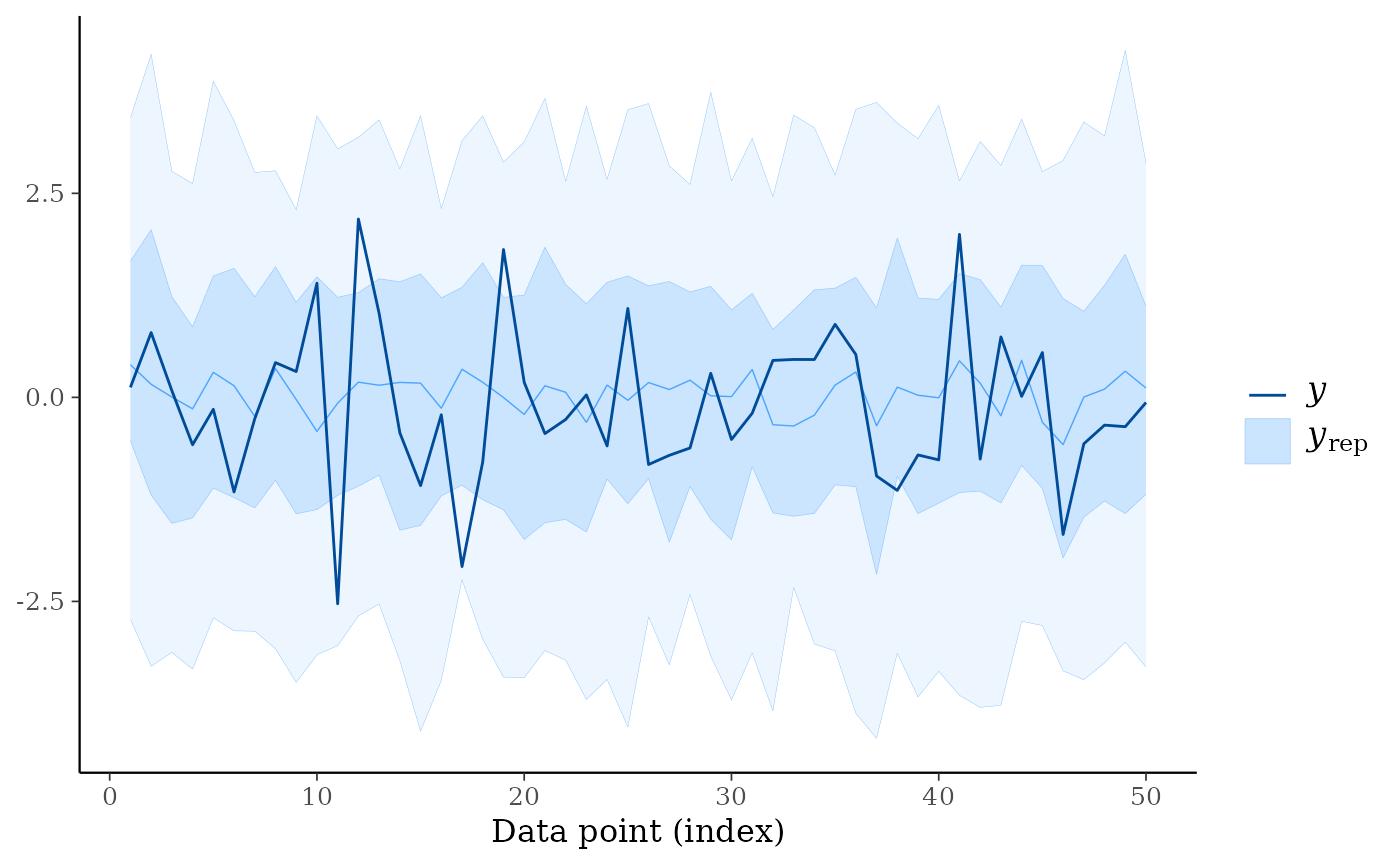# change x axis to y values (instead of indices) and add x = y line
ppc_intervals(y, yrep, x = y) + abline_01()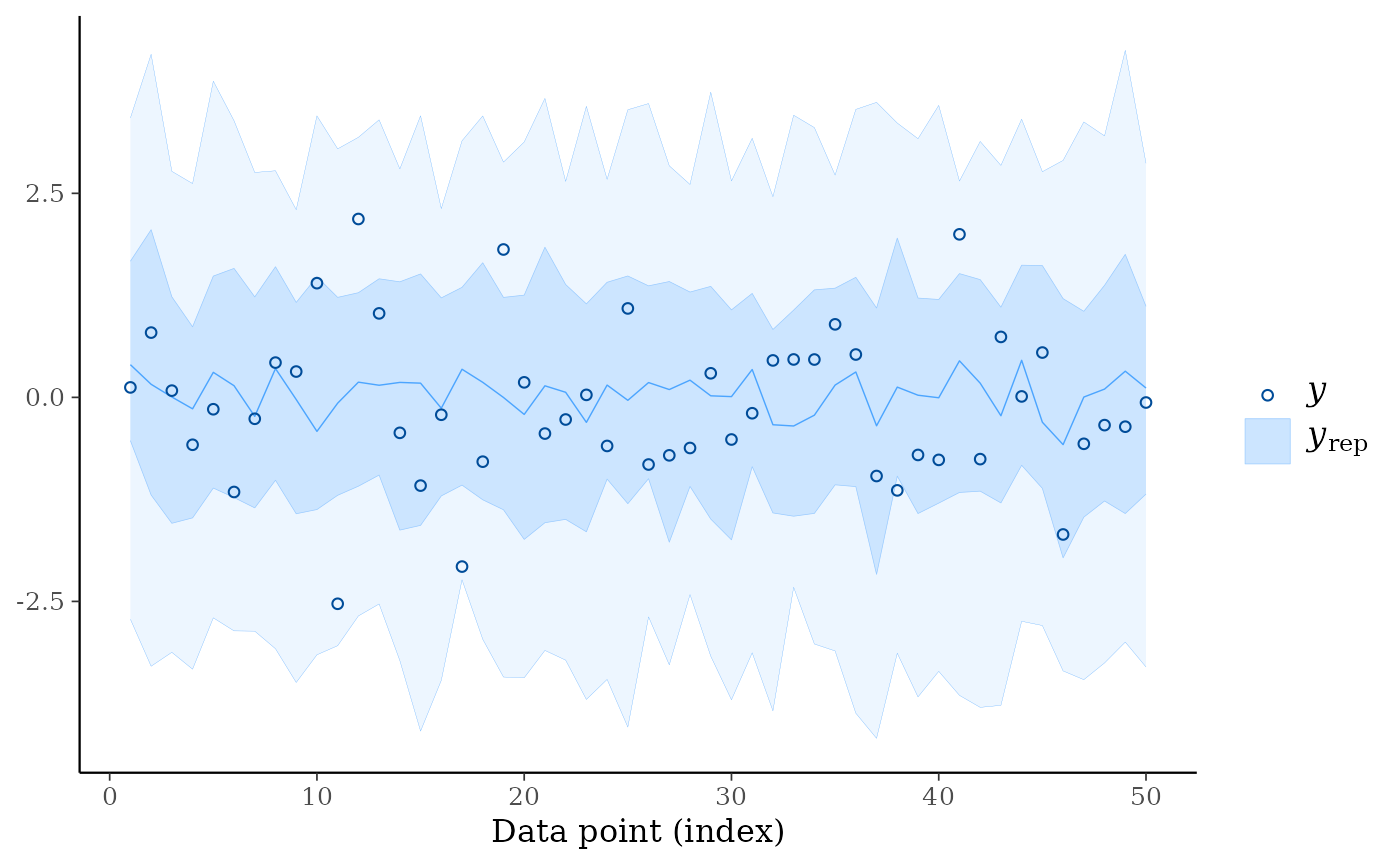color_scheme_set("teal")
year <- 1950:1999
ppc_ribbon(y, yrep, x = year, alpha = 0, size = 0.75) + ggplot2::xlab("Year")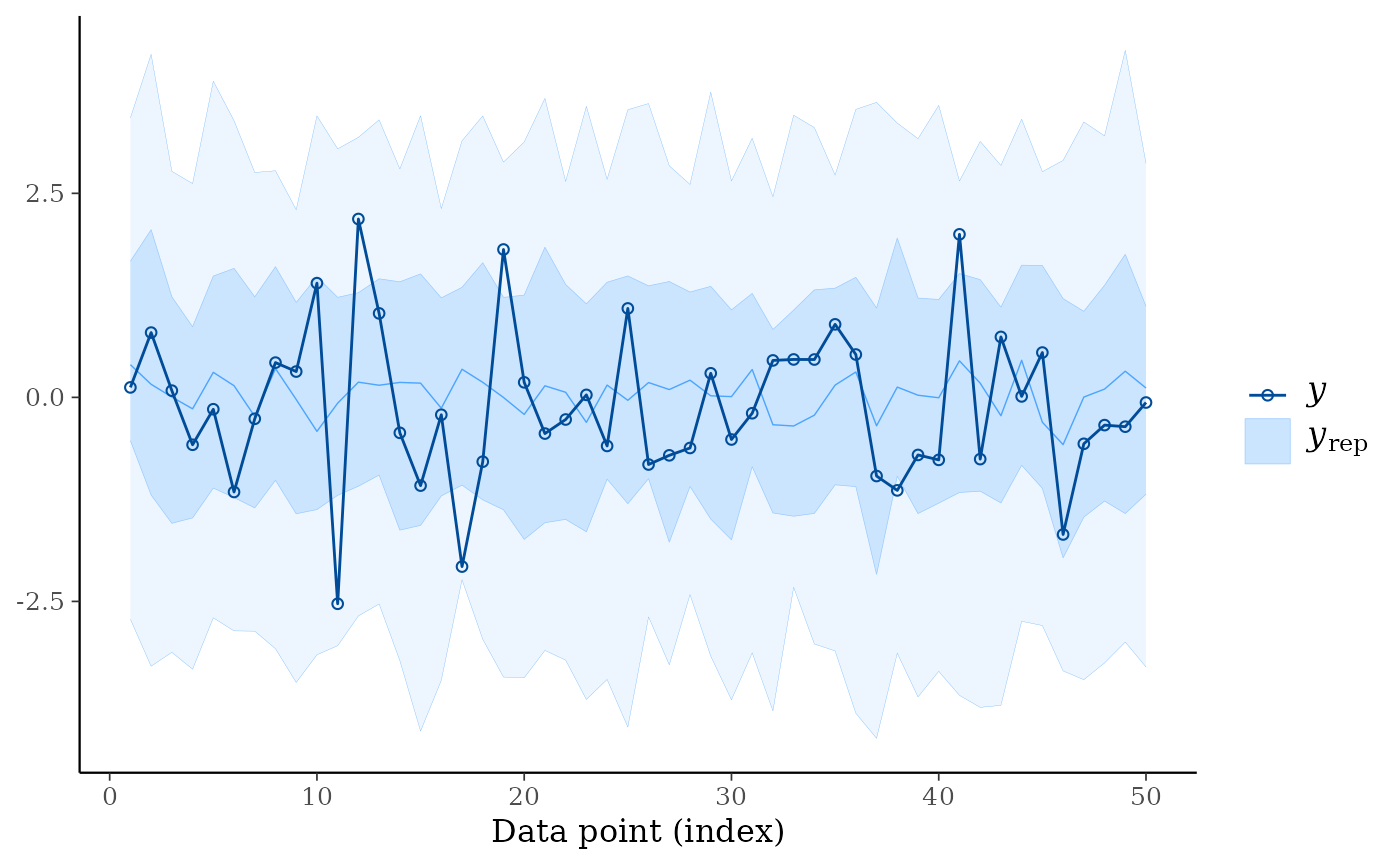color_scheme_set("pink")
year <- rep(2000:2009, each = 5)
group <- gl(5, 1, length = 50, labels = LETTERS[1:5])
ppc_ribbon_grouped(y, yrep, x = year, group) +
ggplot2::scale_x_continuous(breaks = pretty)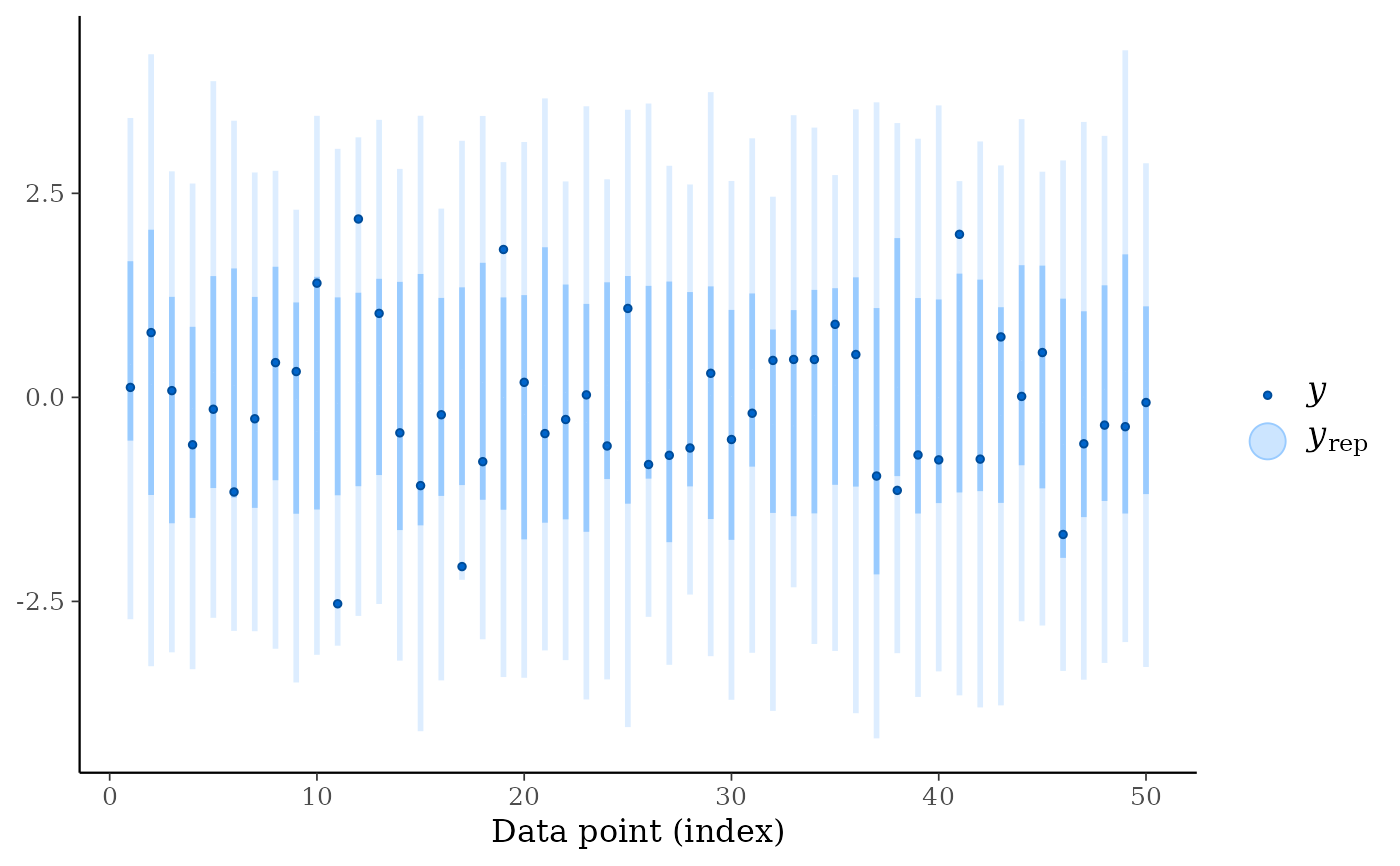ppc_ribbon_grouped(
y, yrep, x = year, group,
facet_args = list(scales = "fixed"),
alpha = 1,
size = 2
) +
xaxis_text(FALSE) +
xaxis_ticks(FALSE) +
panel_bg(fill = "gray20")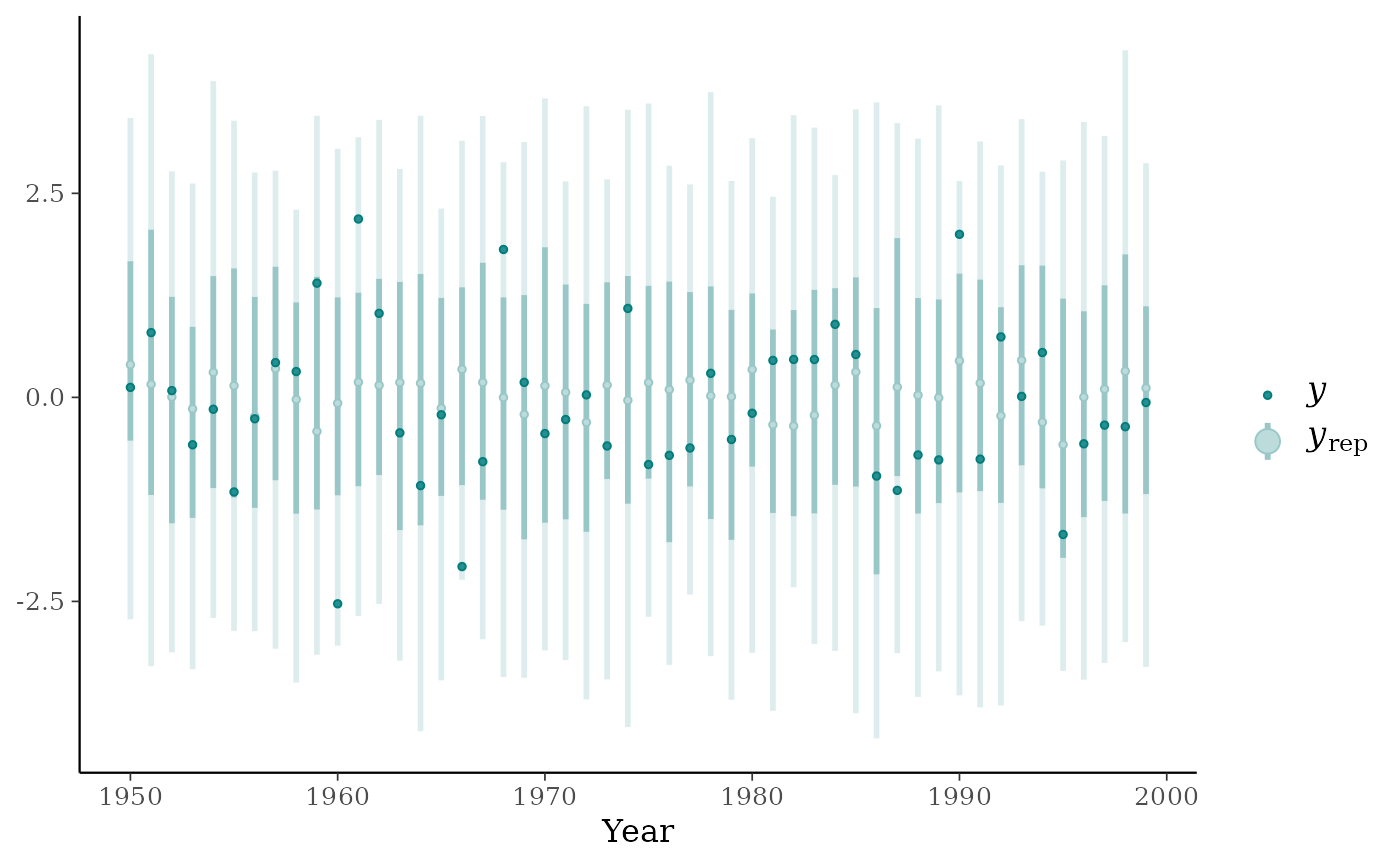ppc_dat <- ppc_intervals_data(y, yrep, x = year, prob = 0.5)
ppc_group_dat <- ppc_intervals_data(y, yrep, x = year, group = group, prob = 0.5)library("rstanarm")
fit <- stan_glmer(mpg ~ wt + (1|cyl), data = mtcars, refresh = 0)#> Warning: There were 1 divergent transitions after warmup. Increasing adapt_delta above 0.95 may help. See
#> http://mc-stan.org/misc/warnings.html#divergent-transitions-after-warmup#> Warning: Examine the pairs() plot to diagnose sampling problemsyrep <- posterior_predict(fit)

color_scheme_set("purple")
with(mtcars, ppc_intervals(mpg, yrep, x = wt, prob = 0.5)) +
panel_bg(fill="gray90", color = NA) +
grid_lines(color = "white")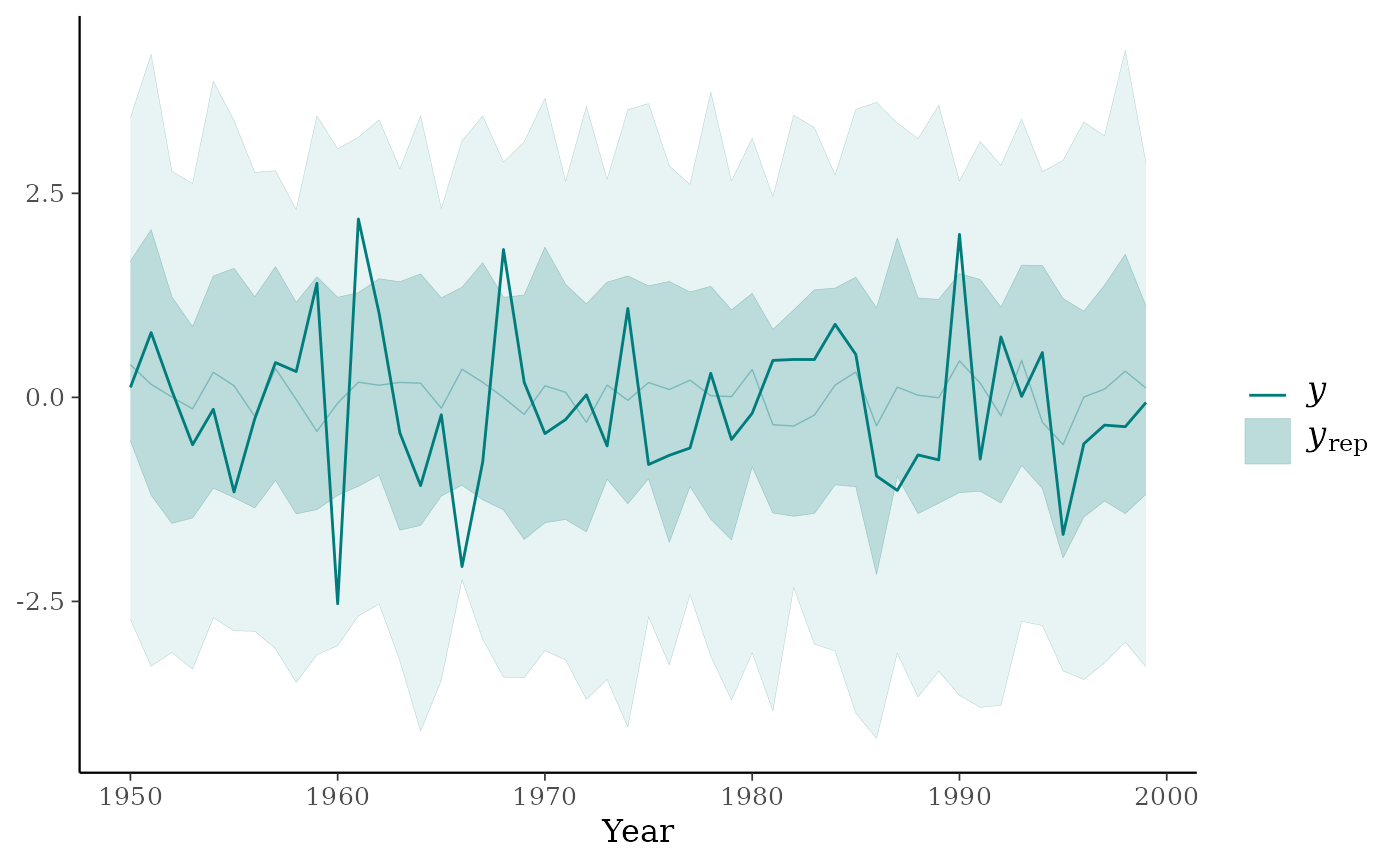ppc_intervals_grouped(y = mtcars$mpg, yrep, prob = 0.8, x = mtcars$wt, group = mtcars$cyl)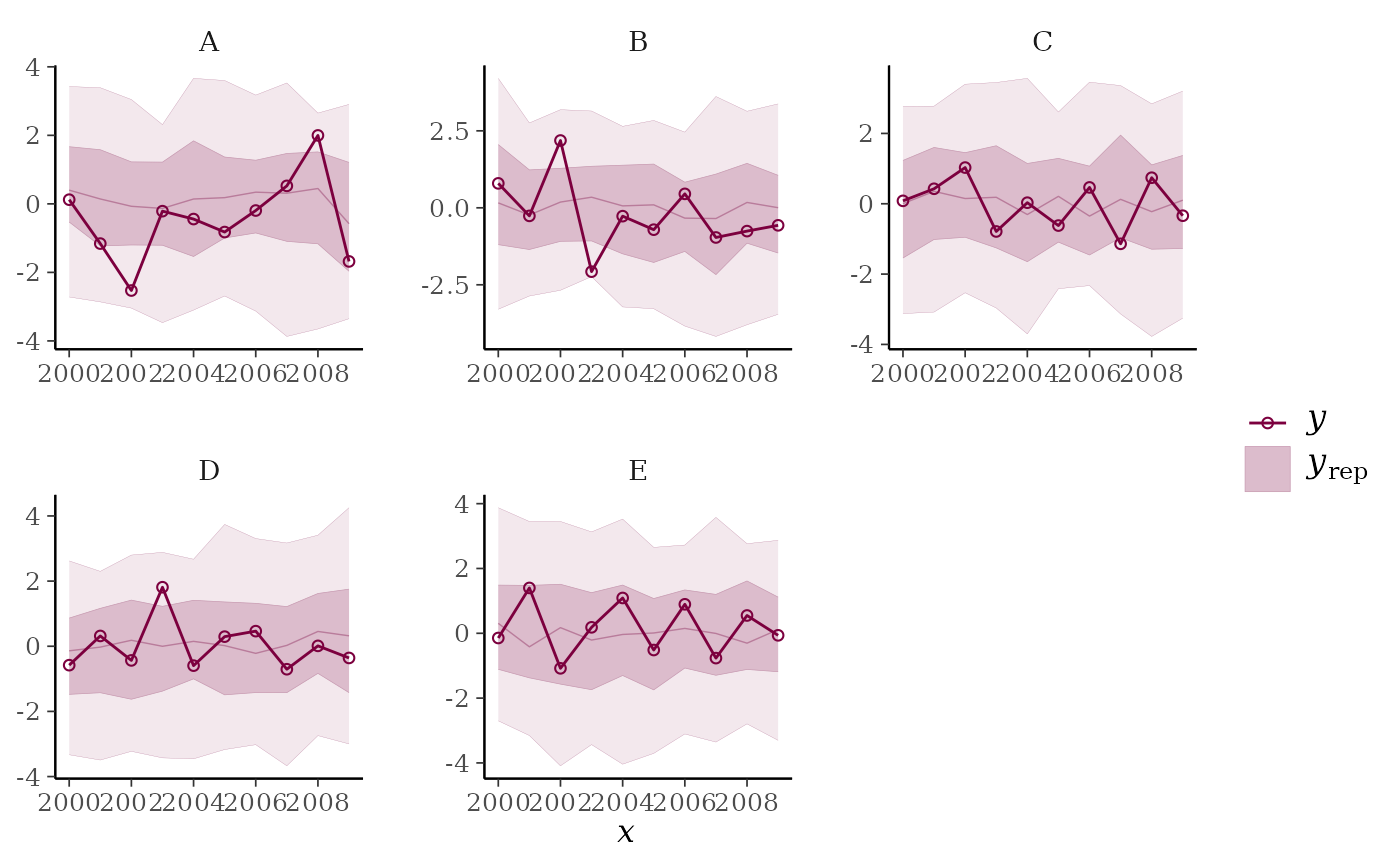color_scheme_set("gray") ppc_intervals(mtcars$mpg, yrep, prob = 0.5) +
ggplot2::scale_x_continuous(
labels = rownames(mtcars),
breaks = 1:nrow(mtcars)
) +
xaxis_text(angle = -70, vjust = 1, hjust = 0)